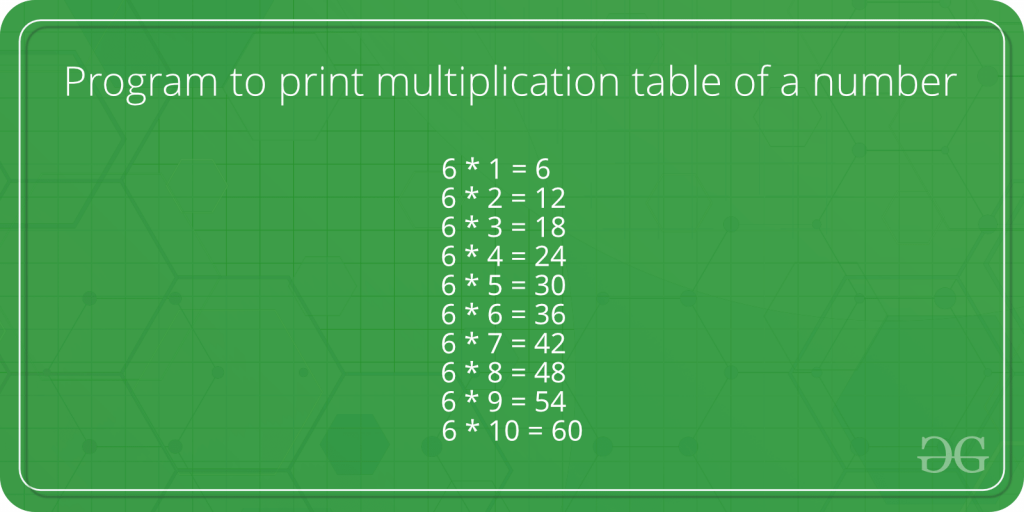# Program to print multiplication table of a number

• Difficulty Level : Basic
• Last Updated : 15 Apr, 2021

Given a number n as input, we need to print its table.Examples :

```Input :  5
Output : 5 * 1 = 5
5 * 2 = 10
5 * 3 = 15
5 * 4 = 20
5 * 5 = 25
5 * 6 = 30
5 * 7 = 35
5 * 8 = 40
5 * 9 = 45
5 * 10 = 50

Input :  8
Output : 8 * 1 = 8
8 * 2 = 16
8 * 3 = 24
8 * 4 = 32
8 * 5 = 40
8 * 6 = 48
8 * 7 = 56
8 * 8 = 64
8 * 9 = 72
8 * 10 = 80
8 * 11 = 88
8 * 12 = 96```

Example 1: Display Multiplication table up to 10

## C++

 `// CPP program to print table of a number``#include ``using` `namespace` `std;` `int` `main()``{``    ``int` `n = 5;  ``// Change here to change output``    ``for` `(``int` `i = 1; i <= 10; ++i)``        ``cout << n << ``" * "` `<< i << ``" = "` `             ``<< n * i << endl;``   ` `    ``return` `0;``}`

## Java

 `// Java program to print table``// of a number``import` `java.io.*;` `class` `table``{  ``    ``// Driver code``    ``public` `static` `void` `main(String arg[])``    ``{  ``        ``// Change here to change output``        ``int` `n = ``5``;``        ` `        ``for` `(``int` `i = ``1``; i <= ``10``; ++i)``            ``System.out.println(n + ``" * "` `+ i +``                               ``" = "` `+ n * i);``    ``}``}` `// This code is contributed by Anant Agarwal.`

## Python

 `# Python Program to print table``# of a number upto 10` `def` `table(n):``    ``for` `i ``in` `range` `(``1``, ``11``):``        ` `        ``# multiples from 1 to 10``        ``print` `"%d * %d = %d"` `%` `(n, i, n ``*` `i)` `# number for which table is evaluated``n ``=` `5``table(n)` `# This article is contributed by Shubham Rana`

## C#

 `// C# program to print``// table of a number``using` `System;` `class` `GFG``{``    ``// Driver code``    ``public` `static` `void` `Main()``    ``{``        ``// Change here to``        ``// change output``        ``int` `n = 5;``        ` `        ``for` `(``int` `i = 1; i <= 10; ++i)``            ``Console.Write(n + ``" * "` `+ i +``                              ``" = "` `+ n *``                               ``i + ``"\n"``);``    ``}``}` `// This code is contributed``// by Smitha.`

## PHP

 ``

## Javascript

 ``

Output :

```5 * 1 = 5
5 * 2 = 10
5 * 3 = 15
5 * 4 = 20
5 * 5 = 25
5 * 6 = 30
5 * 7 = 35
5 * 8 = 40
5 * 9 = 45
5 * 10 = 50```

This program above computes the multiplication table up to 10 only.
The program below is the modification of above program in which the user is also asked to entered the range up to which multiplication table should be displayed.
Example 2: Display multiplication table up to a given range

## C++

 `// CPP program to print table over a range.``#include ``using` `namespace` `std;``int` `main()``{``    ``int` `n = 8;   ``// Change here to change input number``    ``int` `range = 12; ``// Change here to change result.``    ``for` `(``int` `i = 1; i <= range; ++i)``        ``cout << n << ``" * "` `<< i << ``" = "``            ``<< n * i << endl;``    ``return` `0;``}`

## Java

 `// Java program to print table``// over given range.``import` `java.io.*;` `class` `table``{  ``    ``// Driver code``    ``public` `static` `void` `main(String arg[])``    ``{  ``        ``// Change here to change input number``        ``int` `n = ``8``;``        ` `        ``// Change here to change result``        ``int` `range = ``12``;``        ` `        ``for` `(``int` `i = ``1``; i <= range; ++i)``            ``System.out.println(n + ``" * "` `+ i``                             ``+ ``" = "` `+ n * i);``    ``}``}` `// This code is contributed by Anant Agarwal.`

## Python

 `# Python Program to print table``# of a number given range` `def` `table(n, r):``    ``for` `i ``in` `range` `(``1``, r ``+` `1``):``        ` `        ``# multiples from 1 to r (range)``        ``print` `"%d * %d = %d"` `%` `(n, i, n ``*` `i)` `# number for which table is evaluated``n ``=` `8` `# range upto which multiples are to be calculated``r ``=` `12``table(n,r)` `# This article is contributed by Shubham Rana`

## C#

 `// C# program to print``// table over given range.``using` `System;` `class` `GFG``{``    ``// Driver code``    ``public` `static` `void` `Main()``    ``{``        ``// Change here to``        ``// change input number``        ``int` `n = 8;``        ` `        ``// Change here to``        ``// change result``        ``int` `range = 12;``        ` `        ``for` `(``int` `i = 1; i <= range; ++i)``            ``Console.Write(n + ``" * "` `+``                          ``i + ``" = "` `+``                          ``n * i + ``"\n"``);``    ``}``}` `// This code is contributed``// by Smitha.`

## PHP

 ``

## Javascript

 ``

Output:

```8 * 1 = 8
8 * 2 = 16
8 * 3 = 24
8 * 4 = 32
8 * 5 = 40
8 * 6 = 48
8 * 7 = 56
8 * 8 = 64
8 * 9 = 72
8 * 10 = 80
8 * 11 = 88
8 * 12 = 96```

This article is contributed by Anurag Rawat. If you like GeeksforGeeks and would like to contribute, you can also write an article using contribute.geeksforgeeks.org or mail your article to contribute@geeksforgeeks.org. See your article appearing on the GeeksforGeeks main page and help other Geeks.